StatLect

# Elementary matrix

An elementary matrix is a square matrix that has been obtained by performing an elementary row or column operation on an identity matrix.## Definition

Remember that there are three types of elementary row operations:

1. interchange two rows;

2. multiply a row by a non-zero constant;

3. add a multiple of one row to another row.

Elementary column operations are defined similarly (interchange, addition and multiplication are performed on columns).

When elementary operations are carried out on identity matrices they give rise to so-called elementary matrices.

Definition Amatrixis said to be an elementary matrix if and only if it is obtained by performing an elementary (row or column) operation on theidentity matrix.

## Examples

Some examples of elementary matrices follow.

Example If we take theidentity matrix and multiply its first row by, we obtain the elementary matrix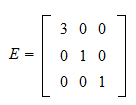Example If we take theidentity matrix and add twice its second column to the third, we obtain the elementary matrix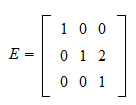Example If we take theidentity matrix and interchange its two columns, we obtain the elementary matrix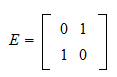## How elementary matrices act on other matrices

As we have already explained, elementary matrices can be used to perform elementary operations on other matrices. The following two procedures are equivalent:

1. perform an elementary operation on a matrix;

2. perform the same operation onand obtain an elementary matrix; pre-multiplybyif it is a row operation, or post-multiplybyif it is a column operation.

## Representation as rank one update

It is possible to represent elementary matrices as rank one updates to the identity matrix.

Proposition Anyelementary matrixcan be written aswhereandare twocolumn vectors and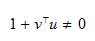Proof

Denote bythecolumns of theidentity matrix(i.e., thevectors of the standard basis). We prove this proposition by showing how to setandin order to obtain all the possible elementary operations. Let us start from row and column interchanges. SetThen,is amatrix whose entries are all zero, except for the following entries: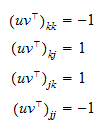As a consequence,is the result of interchanging the-th and-th row of the identity matrix (or the-th and-th column). Note that, so that. Let us now find how to multiply a row or a column by a non-zero constant. Set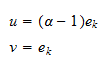Then,is amatrix whose entries are all zero, except for one entry: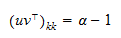As a consequence,is the result of multiplying the-th row (or column) by. Note that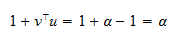which is different from zero becausewas assumed to be. Let us now find how to add a multiple of one row (or column) to another. SetThen,is amatrix whose entries are all zero, except for one entry:Thus,is the result of addingtimes the-th row to the-th (or addingtimes the-th column to the-th). In this case,, so that.

## Invertibility

As we have proved in the lecture on Matrix inversion lemmas, when the conditionis satisfied, rank one updates to the identity matrix are invertible and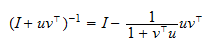Therefore, elementary matrices are always invertible.

Furthermore, the inverse of an elementary matrix is also an elementary matrix. As far as row operations are concerned, this can be seen as follows:

1. ifhas been obtained by multiplying a row of the identity matrix by a non-zero constant, thenis computed by multiplying the same row of the identity matrix by the reciprocal of that constant;

2. ifhas been obtained by adding a multiple of rowto rowof the identity matrix, thenis calculated by subtracting the same multiple of rowfrom rowof the identity matrix;

3. ifis the result of interchanging rowsandof the identity matrix, thenis obtained by interchanging the same rows of the identity matrix again.

Similar statements are valid for column operations (we just need to replace rows with columns in the three points above).